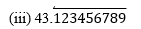Guru

# The following real numbers have decimal expansions as given below. In each case, decide whether they are rational or not. If they are rational, and of the form, p q what can you say about the prime factors of q? (i) 43.123456789 (ii) 0.120120012000120000. . .

• 0

How can i solve this tough question of real numbers . I don’t know the solution of this question. It’s looking tough. So please help for solving this question The following real numbers have decimal expansions as given below. In each case, decide whether they are rational or not. If they are rational, and of the form, p q what can you say about the prime factors of q? (i) 43.123456789 (ii) 0.120120012000120000. . .

Share

1. (i) 43.123456789

Since it has a terminating decimal expansion, it is a rational number in the form of p/q and q has factors of 2 and 5 only.

(ii) 0.120120012000120000. . .

Since, it has non-terminating and non- repeating decimal expansion, it is an irrational number.Since it has non-terminating but repeating decimal expansion, it is a rational number in the form of p/q and q has factors other than 2 and 5.

• 1